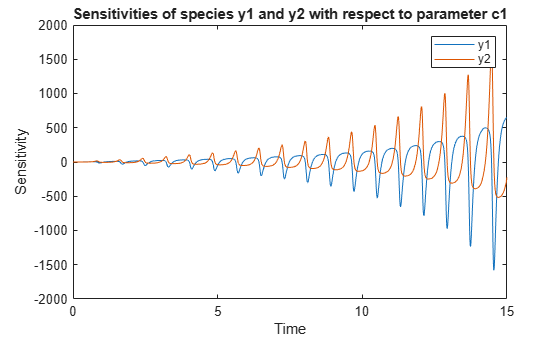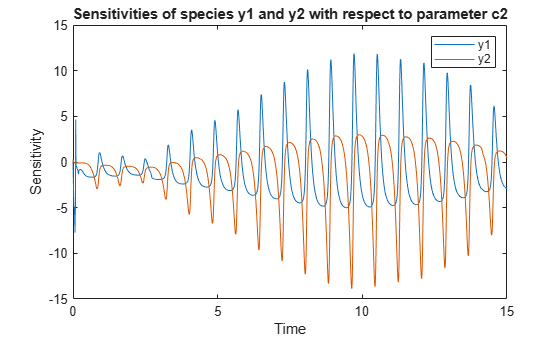SimFunctionSensitivity object

SimFunctionSensitivity object, subclass of SimFunction object

Description

The SimFunctionSensitivity object is a subclass of SimFunction object. It allows you to compute sensitivity.

Syntax

The SimFunctionSensitivity object shares all syntaxes of the SimFunction object. It has the following additional syntax.

[T,Y,SensMatrix] = F(___) returns T, a cell array of numeric vector, Y, a cell array of 2-D numeric matrices, and SensMatrix, a cell array of 3-D numeric matrix containing calculated sensitivities of model quantities. SensMatrix contains a matrix of size TimePoints x Outputs x Inputs. TimePoints is the total number of time points, Outputs is the total number of output factors, and Inputs is the total number of input factors.

If you specify a single output argument, the object returns an SimData object or array of SimData objects with sensitivity information.

Properties

The SimFunctionSensitivity object shares all properties of the SimFunction object. It has the following additional properties.

 SensitivityOutputs table with variables named: 'Name''Type''Units' (only if UnitConversion is turned on)This table contains information about model quantities (species or parameters) for which you want to compute the sensitivities. Sensitivity output factors are the numerators of time-dependent derivatives described in Sensitivity Analysis in SimBiology. This property is read only. SensitivityInputs table with variables named: 'Name''Type''Units' (only if UnitConversion is turned on)This table contains information about model quantities (species, compartments, or parameters) with respect to which you want to compute the sensitivities. Sensitivity input factors are the denominators of time-dependent derivatives described in Sensitivity Analysis in SimBiology. This property is read only. SensitivityNormalization Character vector specifying the normalization method for calculated sensitivities. The following examples show how sensitivities of a species x with respect to a parameter k are calculated for each normalization type.'None' — No normalization. $\frac{\partial x\left(t\right)}{\partial k}$'Half' — Normalization relative to the numerator only. $\left(\frac{1}{x\left(t\right)}\right)\left(\frac{\partial x\left(t\right)}{\partial k}\right)$'Full' — Full dedimensionalization $\left(\frac{k}{x\left(t\right)}\right)\left(\frac{\partial x\left(t\right)}{\partial k}\right)$

Examples

collapse all

This example shows how to calculate the sensitivities of some species in the Lotka-Volterra model using the SimFunctionSensitivity object.

Define the input parameters.

params = {'Reaction1.c1', 'Reaction2.c2'};

Define the observed species, which are the outputs of simulation.

observables  = {'y1', 'y2'};

Create a SimFunctionSensitivity object. Set the sensitivity output factors to all species (y1 and y2) specified in the observables argument and input factors to those in the params argument (c1 and c2) by setting the name-value pair argument to 'all'.

f = createSimFunction(m1,params,observables,[],'SensitivityOutputs','all','SensitivityInputs','all','SensitivityNormalization','Full')
f =
SimFunction

Parameters:

Name          Value        Type
________________    _____    _____________

{'Reaction1.c1'}      10     {'parameter'}
{'Reaction2.c2'}    0.01     {'parameter'}

Observables:

Name        Type
______    ___________

{'y1'}    {'species'}
{'y2'}    {'species'}

Dosed: None

Sensitivity Input Factors:

Name              Type
________________    _____________

{'Reaction1.c1'}    {'parameter'}
{'Reaction2.c2'}    {'parameter'}

Sensitivity Output Factors:

Name        Type
______    ___________

{'y1'}    {'species'}
{'y2'}    {'species'}

Sensitivity Normalization:

Full

Calculate sensitivities by executing the object with c1 and c2 set to 10 and 0.1, respectively. Set the output times from 1 to 10. t contains time points, y contains simulation data, and sensMatrix is the sensitivity matrix containing sensitivities of y1 and y2 with respect to c1 and c2.

[t,y,sensMatrix] = f([10,0.1],[],[],1:10);

Retrieve the sensitivity information at time point 5.

temp = sensMatrix{:};
sensMatrix2 = temp(t{:}==5,:,:);
sensMatrix2 = squeeze(sensMatrix2)
sensMatrix2 = 2×2

37.6987   -6.8447
-40.2791    5.8225

The rows of sensMatrix2 represent the output factors (y1 and y2). The columns represent the input factors (c1 and c2).

$sensMatrix2=\left[\begin{array}{cc}\begin{array}{c}\frac{\partial y1}{\partial c1}\\ \\ \frac{\partial y2}{\partial c1}\end{array}& \begin{array}{c}\frac{\partial y1}{\partial c2}\\ \\ \frac{\partial y2}{\partial c2}\end{array}\end{array}\right]$

Set the stop time to 15, without specifying the output times. In this case, the output times are the solver time points by default.

sd = f([10,0.1],15);

Retrieve the calculated sensitivities from the SimData object sd.

[t,y,outputs,inputs] = getsensmatrix(sd);

Plot the sensitivities of species y1 and y2 with respect to c1.

figure;
plot(t,y(:,:,1));
legend(outputs);
title('Sensitivities of species y1 and y2 with respect to parameter c1');
xlabel('Time');
ylabel('Sensitivity');Plot the sensitivities of species y1 and y2 with respect to c2.

figure;
plot(t,y(:,:,2));
legend(outputs);
title('Sensitivities of species y1 and y2 with respect to parameter c2');
xlabel('Time');
ylabel('Sensitivity');Alternatively, you can use sbioplot.

sbioplot(sd);You can also plot the sensitivity matrix using the time integral for the calculated sensitivities of y1 and y2. The plot indicates y1 and y2 are more sensitive to c1 than c2.

[~, in, out] = size(y);
result = zeros(in, out);
for i = 1:in
for j = 1:out
result(i,j) = trapz(t(:),abs(y(:,i,j)));
end
end
figure;
hbar = bar(result);
haxes = hbar(1).Parent;
haxes.XTick = 1:length(outputs);
haxes.XTickLabel = outputs;
legend(inputs,'Location','NorthEastOutside');
ylabel('Sensitivity');